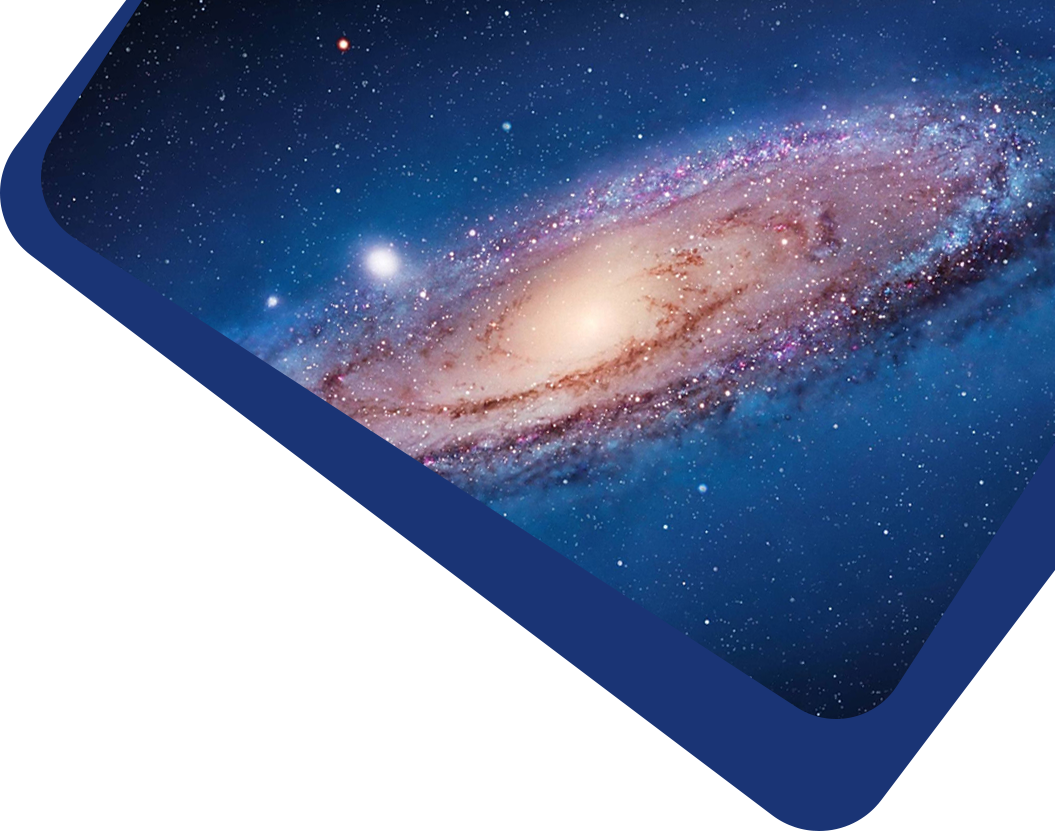Vol 21, No 9

## An Earth-mass planet in a time of COVID-19: KMT-2020-BLG-0414Lb

#### Wei-Cheng Zang, Cheongho Han, Iona Kondo, Jennifer C. Yee, Chung-Uk Lee, Andrew Gould, Shude Mao, Leandro de Almeida, Yossi Shvartzvald, Xiang-Yu Zhang, Michael D. Albrow, Sun-Ju Chung, Kyu-Ha Hwang, Youn Kil Jung, Yoon-Hyun Ryu, In-Gu Shin, Sang-Mok Cha, Dong-Jin Kim, Hyoun-Woo Kim, Seung-Lee Kim, Dong-Joo Lee, Yongseok Lee, Byeong-Gon Park, Richard W. Pogge, John Drummond, Thiam-Guan Tan, Josè Dias do Nascimento Júnior, Dan Maoz, Matthew T. Penny, Wei Zhu, Ian A. Bond, Fumio Abe, Richard Barry, David P. Bennett, Aparna Bhattacharya, Martin Donachie, Hirosane Fujii, Akihiko Fukui, Yuki Hirao, Yoshitaka Itow, Rintaro Kirikawa, Naoki Koshimoto, Man Cheung Alex Li, Yutaka Matsubara, Yasushi Muraki, Shota Miyazaki, Greg Olmschenk, Clément Ranc, Nicholas J. Rattenbury, Yuki Satoh, Hikaru Shoji, Stela Ishitani Silva, Takahiro Sumi, Daisuke Suzuki, Yuzuru Tanaka, Paul J. Tristram, Tsubasa Yamawaki, Atsunori Yonehara, Andreea Petric, Todd Burdullis, Pascal Fouqué

Abstract

Abstract We report the discovery of KMT-2020-BLG-0414Lb, with a planet-to-host mass ratio $$q_2 = 0.9 - 1.2 \times 10^{-5} = 3-4 q_{\bigoplus}$$ at 1σ, which is the lowest mass-ratio microlensing planet to date. Together with two other recent discoveries ($$4\lesssim q/q_{\bigoplus} \lesssim 6$$), it fills out the previous empty sector at the bottom of the triangular (log $$s$$, log $$q$$) diagram, where $$s$$ is the planet-host separation in units of the angular Einstein radius $$\theta_{\rm E}$$. Hence, these discoveries call into question the existence, or at least the strength, of the break in the mass-ratio function that was previously suggested to account for the paucity of very low-$$q$$ planets. Due to the extreme magnification of the event, $$A_{\rm max}\sim 1450$$ for the underlying single-lens event, its light curve revealed a second companion with $$q_3 \sim 0.05$$ and $$| \log s_3| \sim 1$$, i.e., a factor $$\sim$$ 10 closer to or farther from the host in projection. The measurements of the microlens parallax $$\pi_{\rm E}$$ and the angular Einstein radius $$\theta_{\rm E}$$ allow estimates of the host, planet and second companion masses, $$(M_1, M_2, M_3) \sim (0.3 M_{\odot}, 1.0 M_{\bigoplus}, 17 M_J)$$, the planet and second companion projected separations, $$(a_{\perp,2}, a_{\perp,3}) \sim$$ (1.5, 0.15 or 15) au, and system distance $$D_{\rm L} \sim 1$$ kpc. The lens could account for most or all of the blended light ($$I \sim 19.3$$) and so can be studied immediately with high-resolution photometric and spectroscopic observations that can further clarify the nature of the system. The planet was found as part of a new program of high-cadence follow-up observations of high-magnification events. The detection of this planet, despite the considerable difficulties imposed by COVID-19 (two KMT sites and OGLE were shut down), illustrates the potential utility of this program.

Keywords

Keywords planets and satellites: detection, gravitational lensing: micro, (stars:) brown dwarfs, planets and satellites: terrestrial planets

Full Text
Refbacks

• There are currently no refbacks.